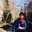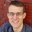Question-and-Answer Resource for the Building Energy Modeling Community
Get s tarted with the Help page

# Energy simulation without geometryHi all,

I want to do preliminary calculation how much energy will be consumed just inputting following parameter

• Lighting Power Density [W/m2]
• Small power [W/m2]
• Zone area [m2]
• Schedule

Since the HVAC consumption is not critical in this project, we want to skip the procedure to construct the geometry and just input area information to get preliminary result.
Are there any example file or page I can refer to how to do that?

edit retag close merge delete

1

Why is a simulation necessary? You can do it with Excel.

Sort by » oldest newest most votedSchedules can be translated into a singular "Full Load Hour" value. If you multiply your full load value times the full load hours, it equals the sum of each fractional value times each hour.

For example, if your schedule fraction is 0.3 for 24 hours, then your daily full load hours are 0.3 * 24 hrs = 7.2 full load hours. Or if you have 12 hours at 0.3 , and 12 hours at 0.5, then your full load hours are 0.3 * 12 hrs + 0.5 * 12 hrs = 9.6 full load hours.

You can calculate the full load hours in a year for a given schedule, which will typically be between 2,000 - 5,000 hours for lighting and plug loads. See the "EFLH worksheet" in the LEED performance calculator to calculate annual Equivalent Full Load Hours for a given schedule.

You can then calculate annual energy use = (Lighting Power Density [W/m2]) * (Zone area [m2]) * (Lighting Schedule annual EFLH) + (Small Power Density [W/m2]) * (Zone area [m2]) * (Small Power Schedule annual EFLH)

more